﻿ Quantization of Harmonic Oscillator Soliton by Friction Term MethodInternational Journal of Theoretical and Mathematical Physics

p-ISSN: 2167-6844    e-ISSN: 2167-6852

2018;  8(4): 89-93

doi:10.5923/j.ijtmp.20180804.02### Quantization of Harmonic Oscillator Soliton by Friction Term Method

Isam Ahmed Attia1, Mubarak Dirar AbdAlla1, Rasha Abdelhai Mohamed Taha1, 2

1Physics Department, College of Science, Sudan University of Science & Technology, Khartoum, Sudan

2Physics Department, College of Science, Majmaah University, Zulfi, Saudi Arabia

Correspondence to: Isam Ahmed Attia, Physics Department, College of Science, Sudan University of Science & Technology, Khartoum, Sudan.
 Email: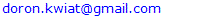Abstract

We derive results for two solitons solutions of the motion of a one-dimensional harmonic oscillator with frictional term and use them to obtain two describe different solitons, one represents exponentially increasing beam, while the other stand for exponentially decreasing beam. The Hamiltonian corresponding to these harmonic oscillator solitons lead to describe some physical systems. We could, however, make use of theoretic approach to quantize the classical motion using Hamiltonian, temperature, relaxation time, and crystal Potential, and thus, demonstrate that the corresponding quantum systems are entirely different.

Keywords: Harmonic Oscillator, Soliton, Friction, Quantization, Hamiltonian

Cite this paper: Isam Ahmed Attia, Mubarak Dirar AbdAlla, Rasha Abdelhai Mohamed Taha, Quantization of Harmonic Oscillator Soliton by Friction Term Method, International Journal of Theoretical and Mathematical Physics, Vol. 8 No. 4, 2018, pp. 89-93. doi: 10.5923/j.ijtmp.20180804.02.

### 1. Introduction

Solitons in contemporary understanding were first described by N.J. Zabusky and M.D. Kruskal  in 1965 and they form now a paradigm in mathematical physics (see also [2, 3, 4]). Soliton is a solitary wave with finite energy and the necessary conditions of its existence include nonlinearity and dispersion. Soliton dynamics is one of the hot topics due to wide applications in hydrodynamics, electronics, solid mechanics, biophysics and other disciplines, and accompanying theoretical deepness (Ablowitz and Clarkson 1991, Fokas and Zakharov 1993). They dealt with the dynamics of one-dimensional (1D) anharmonic lattices and their (quasi) continuum approximation  provided by the Boussinesq–Korteweg-de Vries equation [6, 7, 8]. That work followed research done by Schrödinger, when he given the Nonlinear Schrödinger Equation (NLSE) [9, 10] Appears in Various Physical Contexts to describe the propagation of nonlinear waves .
The significant achievements of the sine-Gordon (SG) equation, The SG equation appears in a number of physical applications, including dislocations in the crystals and the movement of a rigid pendulum attached to a stretched wire . Of more, the SG equation can be used to describe elementary particles and the propagation of ultra-short optical pulses in lasers.
While trying to understand soliton, they used the mathematical aspects of the quantum friction instabilities are manifested in the fact that the system may support natural modes of oscillation that grow exponentially with time [13, 14, 15], even in presence of system loss . In this article, we establish definite link between this classical effect and quantum friction for harmonic oscillator soliton.
Very interestingly, the harmonic oscillator comprises one of the most important examples of elementary Quantum Mechanics. There are several reasons for its pivotal role. The linear harmonic oscillator describes vibrations in molecules and their counterparts in solids, the phonons. Many more physical systems can, at least approximately, be described in terms of linear harmonic oscillator models. However, the most eminent role of this oscillator is its linkage to the electron, one of the conceptual building blocks of microscopic physics. For example, electron describe the modes of the radiation field, providing the basis for its quantization .

### 2. Theoretical Model (Harmonic Oscillator Soliton)

In non-relativistic quantum mechanics, a particle (such as an electron or proton) is described by a complex wave-function,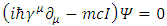whose time evolution is governed by the Schrödinger equation :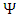(1)
where 𝑖 is the imaginary number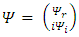is the reduced Planck constant which is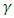is the wave function of the quantum system, x is the position in a one-dimensional coordinate system, and t the time.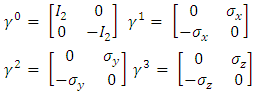is the Hamiltonian operator (which characterizes the total energy of the system under consideration).
The classical harmonic oscillator is most frequently introduced as a mass on an undamped spring. The Hamiltonian for such a system is: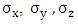(2)
where k is the force constant for the Harmonic oscillator. (Note: the k here has nothing to do with momentum eigenvalues. It is just coincidental that we are using the same letter in the alphabet to describe these two unrelated items.), m is mass of a particle, the Hamiltonian above describes ellipses in phase space: this is the classical motion of harmonic oscillators. Both the position x and the momentum p vary with time harmonically, hence the name. second term in equation (2) stand for the potential of the restoring force.
As is well known, quantum systems are not in rest at zero temperature, which manifests itself by the non-zero vacuum energy. Hence, according to the previous studies the interaction of an electron, for instance, with a frozen classical surrounding will result in appearance of a quantum friction. This effect should be also present even in atoms and molecules, where the contemporary quantum mechanics does not consider, surprisingly, any collisions between the building particles. Since the electrons are negatively charged, the electron-electron collisions are probably very rare events, but they could be important for electron transitions in atoms and molecules.  The collisions between an electron and relatively large positive nuclei seem, however, inevitable unless it is restricted by relativistic effects.
Now, if the Harmonic oscillator has resistive force of friction where the friction force is inversely proportional to the relaxation time . and by using the relaxation times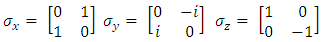, we can turn on a generating frictional energy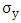(3)
So that we introduce a perturbation term, and the total Hamiltonian then becomes changing. Where the total energy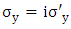is the sum of ordinary free particle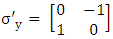beside the frictional one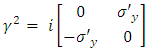which represents here an imaginary part, i.e.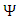(4)
To see the physical meaning of plus and minus sign in equation. consider for resistive medium the wave function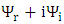is written as: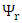(5)
After substituting equation (4) in equation (5) we obtain the equation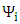(6)
The above wave functions can be written in the form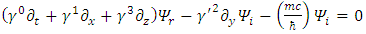(6)
the Probability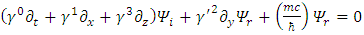of observing the particle in the region (x, x + δx) at time t, given the number of particles at any time t: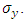(7)
by using equation (3) with plus sign: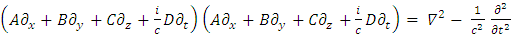(8)
The equation (7) becomes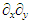(9)
The equation (9) shows that measurement of the number of charges (beam intensity) this equation tells us that the number of particles in a beam grow exponentially with time. This can describe nuclear fission reaction, where the number of neutrons grow and increase with time . The equation (9) can also describe amplification of light , where the number of photons increase with time.
The solutions of the equations of harmonic oscillator with positive friction term obtained persistently point to the regions where the stable soliton-like a small perturbation that effectively increases the soliton power results in an unbounded growth of the soliton amplitude and subsequent beam collapse However, the minus sign of Ef in equation (3) given another physical meaning. To do this consider the minus sign, i.e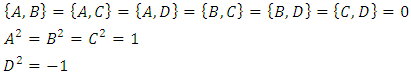(10)
Inserting equation (10) in equation (6), yields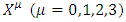(11)
the Probability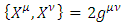of observing the particle in the region (x, x + δx) at time t, given the number of particles at any time t: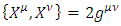(12)
this expiration describes time decaying particles abeam grow exponentially such expiation can be suitable for describe light absorption where that light intensity decreases with time and distance x , by choosing(13)
the intensity given by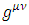(14)
It can also describe neutron absorption, by neutron capture process according to equation(15)
The solutions of the equations of harmonic oscillator with negative friction term obtained persistently point to the regions where the unstable soliton-like,
a small perturbation that effectively decreases the soliton power results in an unbounded decaying of the soliton amplitude and subsequent beam collapse,
a small decrease of the soliton power leads to a switching of a soliton of a stable.
Thus, the plus and minus sign in equation (3) describe different solitons, one represents exponentially increasing beam (7), while the other stand for exponentially decreasing beam (14).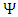Figures 1a,b. We show two different types of the soliton

### 3. Quantization of the Harmonic Oscillator Soliton

Quantization is the process of replacing a range of real numbers by a single number, studies in the quantization of dissipative systems play a role in several areas of physics ranging from electrodynamics to chromodynamics. In spite of this, the problem of quantizing the simplest dissipative system, namely, the harmonic oscillator soliton (HOS).
When the oscillating string is embedded in a resistive matter or crystal, having crystal field of potential Vo the total new Hamiltonian oscillator is given by(16)
Where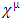(17)
For the Hamiltonian oscillator see equation (16)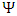(18)
Where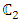Standing for thermal energy and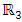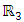(19)
In the momentum space in the ordinary Schrödinger equation in the abstract space is written as: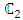(20)
To quantize the oscillator, one replaces the classical variables p and x by the operators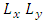and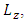In the view of equations (17, 18, 19, 20) and using the fact that in momentum space: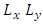(21)
One gets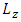(22)
By separating the variables, i.e.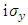(23)
such that one can write the harmonic oscillator equation in the momentum space this can be obtain by equations (1) and (2):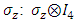(24)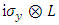(25)
which is solved by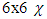(26)
We notice that for simplicity let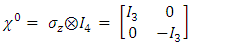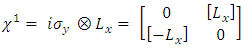(27)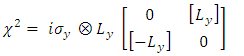(28)
Altogether one can express then the momentum part is given by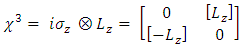(29)
Carrying out the rearranging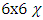(30)
LetThus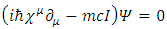(31)
Substitute equation (31) in equation (30)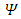(32)
Now let α adjust itself such that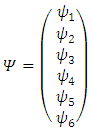(33)
Where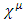(34)
One thus can rearrange equation (32) to get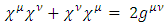(35)
One can solve this equation by assuming solution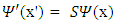(36)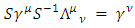(37)
Inserting equation (34) in equation (37) yields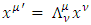(38)
This is where the series method comes in. We can expand the function as a power series, consider new the solution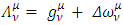(39)
This means that our differential equation is now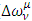(40)
The left side is a polynomial in y. If this is zero for all y, then the overall coefficients are identically zero: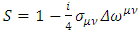(41)
So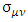(42)
Bearing in mind that the probability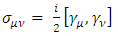is limited, it follows that the summation time in equation (39) is finite, such that the maximum value of (s) is (s=n) this means that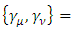(43)
In view of (42) and (43) it follows that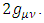(44)
This equation can be satisfied, when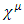(45)
Let us get back into the physics of this. We want to solve the equation with aid of equation (37) one gets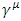(46)
Therefore, the energy eigenvalues are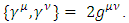(47)
We now have our quantized energies, they are also evenly spaced as expected.
Furthermore, we look at equation (29) gives that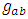It follows that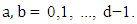(48)
In view of equations (17), (19) and (27), one gets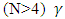(49)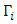(50)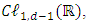(51)
here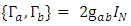are different in the sense that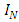stand for classical angular frequency of electron oscillation when only restoring force acts on the electron. The term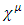however stands for the quantum angular frequency when crystal force, thermal energy force and frictional force in addition to the restoring force act on the electron see equations (34, 18, 19, 20, 24 and 27).
When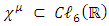only the thermal energy is present, equation (51) reduced to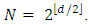(52)
This means that temperature is quantized, where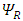(53)
It is shown that the temperature may be quantized in some spacetime, and since the number of particles is (typically) known, the possible temperature values (due to the quantization of energy) can be known exactly.
When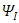the frictional energy is only present, equation (51) read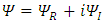(54)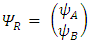(55)
this the relaxation time is quantized, the transport properties of one-dimensional electron systems dependent on relaxation time based on quantum transport processes of spin and charge. One of the most important problems in this matter is conductivity (or resistivity) which should apparently depend on the size of the system. Moreover, we can observe the quantization of conductivity due to geometrical confinement or external magnetic field applied perpendicularly to the plane of motion of electrons.
the same held for the case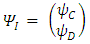when only the crystal potential is present, where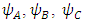(56)
Each electron in an atom has a particular potential energy, where electrons inhabit quantized (discrete) energy states called orbitals, the potential energy V is related to the quantum state, charge, and distance from the nucleus.

### 4. Discussion

Due to string theory, electrons in any atom behaves as a harmonic oscillator. Thus, it is natural to solve the Schrodinger equation for harmonic oscillator in the presence of friction and temperature to be in the form as shown by equations (15-19).
When we are having resistive force (frictional medium), the system generating frictional energy out this change and generate solitary waves (soliton). Thus, after solving the Schrodinger equation for harmonic oscillator in the presence of friction the solitons is formed in a system with two types, one represents exponentially increasing beam (positive frictional term), while the other stand for exponentially decreasing beam (negative frictional term), For the frictional force with the plus sign, the wave function and the number of particles increases with time as shown by equation (7) [22, 23, 25]. This means that amplification can take place. If the frictional force with the minus sign, this expression describes time decaying particles with a beam decaying exponentially, such expression can be suitable to describe light absorption where that having intensity decreases with time and distance x, can also describe neutron absorption, by neutron capture process .
The Hamiltonian in equation (18) represent the total energy for harmonic oscillator soliton, which have four terms equation (49), restoring force energy, thermal energy, the frictional energy, crystal potential.
In Eq. (51) the classical angular frequency of electron oscillation when only restoring force acts on the electron is different from quantum angular frequency when crystal force, thermal energy force and frictional force in addition to the restoring force act on the electron.
In equation (51) When only the thermal energy is greater than other terms, the temperature is quantized (see eq. (53)), When frictional energy is greater than other terms, the relaxation time is quantized (see eq. (54)), the same hold for the case when only the crystal potential is greater than other terms.
Quantization is the process of replacing a range of real numbers by a single number, studies in the quantization of dissipative systems play a role in several areas of physics ranging from electrodynamics to chromodynamics.

### 5. Conclusions

The Schrodinger equation for frictional medium is derived; Harmonic oscillator solitons solutions for resistive medium shows quantization of restoring force energy, temperature, relaxation time and potential crystal.

### References

  N.J. Zabusky and M.D. Kruskal, Phys. Rev. Lett. 15, 57 (1965).  A.C. Scott, F.Y.F. Chu and D.W. McLaughlin, Proc. IEEE 61, 1443 (1973).  A.C. Scott, Nonlinear Science: Emergence & Dynamics of Coherent Structures, 2nd edn. (Oxford University Press, New York, 2003).  N.J. Zabusky, Chaos 15, 015102 (2005).  C.I. Christov, G.A. Maugin and M.G.Velarde, Phys. Rev. E 54, 3621 (1996).  J.V. Boussinesq, Mem. Presented by various scholars at Acad. Sci. Inst. France (Paris), 23, 1 (1877). J.V. Boussinesq, Mem. Presented by scientists to Acad. Sci. Inst. France (Paris), 24, No. 2, 1 (1978).  D.J. Korteweg and G. de Vries, Phil. Mag. 39, 442 (1895).  Richard H. Enns, "It's a Nonlinear World," Springer New York, 2010.  V. Yu.Belashov and S.V. Vladimirov, "Solitary Waves in Dispersive Media Complex", Springer-Verlag Berlin Heidelberg 2005, p-02.  Catherine Sulem and Pierre-Louis Sulem, "The Nonlinear Schrodinger Equation: Self-Focusing and Wave Collapse," Springer-Verlag New York, 1999.  Shekhar Guha and Leonel P. Gonzalez, "Laser Beam Propagation in Nonlinear Optical Media", CRC Press, Taylor & Francis Group, Boca Raton (USA), 2014.  P.G. Drazin, R.S. Johnson, "Solitons: An Introduction", Cambridge University Press (1989).  S. I. Maslovski, M. G. Silveirinha, Quantum friction on monoatomic layers and its classical analogue, Phys. Rev. B, 88, 035427, (2013).  M. G. Silveirinha, “Quantization of the Electromagnetic Field in Non-dispersive Polarizable Moving Media above the Cherenkov Threshold”, Phys. Rev. A, 88, 043846, (2013).  O. Sydoruk, et al, Terahertz instability of surface optical-phonon polaritons that interact with surface plasmon polaritons in the presence of electron drift, Phys. of Plasmas, 17, 102103, (2010).  A. I. Volokitin and B. N. J. Persson, Quantum Friction, Phys. Rev. Lett. 106, 094502 – Published 2 March 2011.  Sophya Garashchuk et al, The Schrödinger equation with friction from the quantum trajectory perspective, THE JOURNAL OF CHEMICAL PHYSICS 138, 054107 (2013).  Pavel Kundrát and Miloš Lokajíček. Three-dimensional harmonic oscillator and time evolution in quantum mechanics. Phys. Rev. A 67, 012104 –13 January 2003. DOI: https://doi.org/10.1103/PhysRevA.67.012104.  Tsekov R and Vayssilov G N 2012 Ann. Phys. (Berlin) 524 822.  Mubarak Dirar et al, Quantum Schrodinger String Theory for Frictional Medium & Collision, International Journal of Innovative Science, Engineering & Technology, Vol. 2 Issue 11, November (2015).  Roumen Tsekov, Quantum friction, Chin. Phys. Lett. 29 (2012) 120504 [arXiv 1203.2421].  Mubarak Dirar et al, String Qunatum Menchical Lasing Due to Friction, American Journal of Innovative Research and Applied Sciences (2016); 3(3): 490-494.  Lutfi Mohammed AbdAlgadir et, Schrodinger Quantum Equation from Classical and Quantum Harmonic Oscillator, International Journal of Engineering Science & Research Technology, (2016).  Amna Al Ata Ahmed Salih et al, Quantum Radioactive Decay Law and Relaxation Time, Journal of Applied Physics, e-ISSN: 2278-4861.Volume 7, Issue 4 Ver. I (Jul. - Aug. (2015), PP 86-89 DOI: 10.9790/4861-07418689.  Ebtisam A. Mohamed, Potential Dependent Frictional Schrodinger Equation, American Scientific Research Journal for Engineering, Technology, and Sciences (ASRJETS) ISSN (Print) 2313-4410, ISSN (Online) 2313-4402.